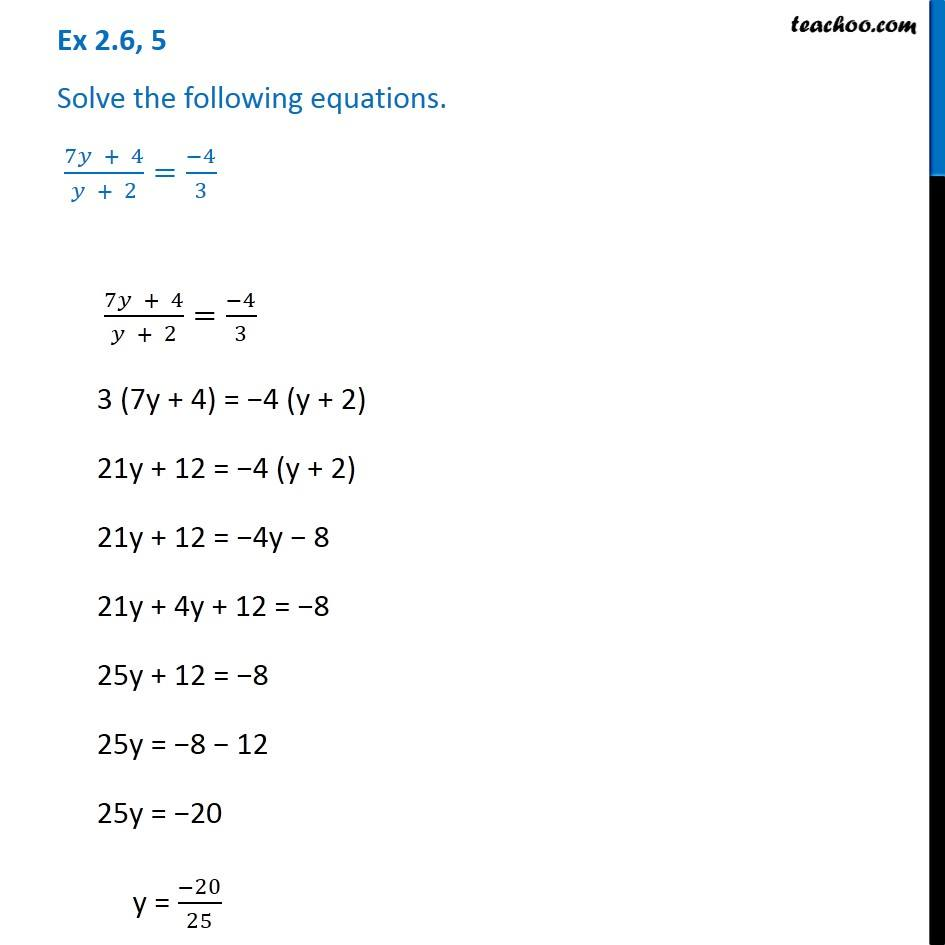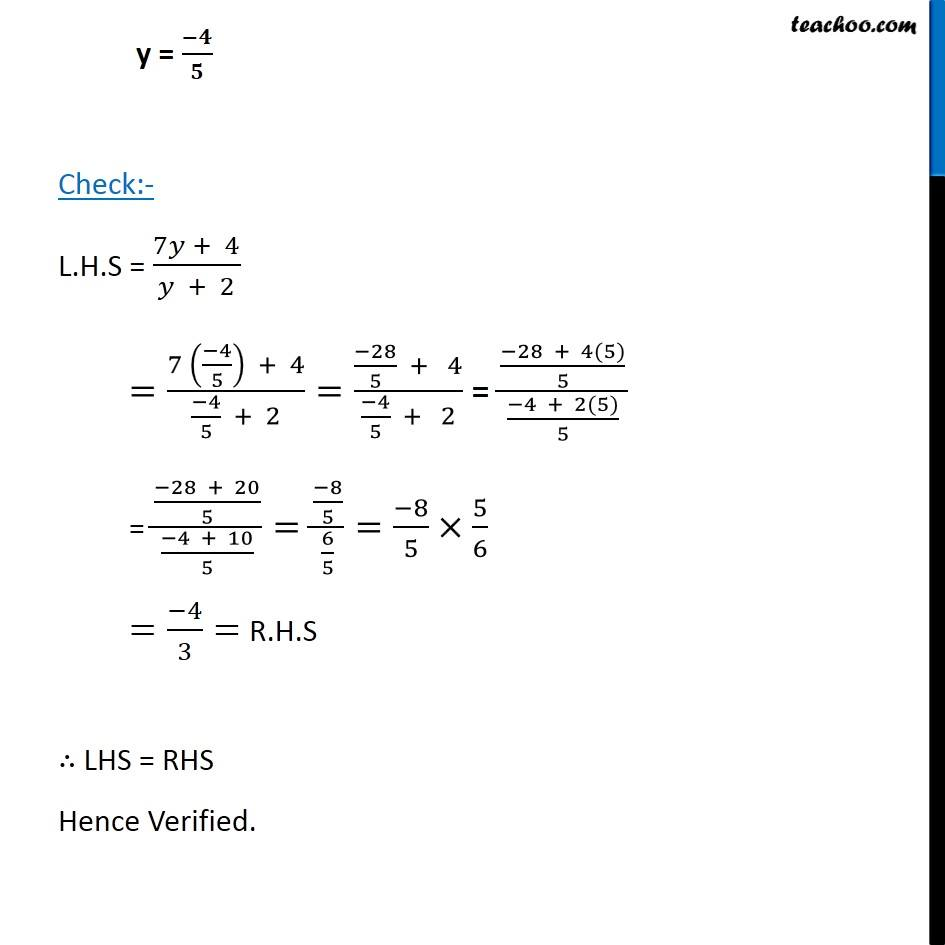Ex 2.6

Chapter 2 Class 8 Linear Equations in One Variable
Serial order wiseGet live Maths 1-on-1 Classs - Class 6 to 12

### Transcript

Ex 2.6, 5 Solve the following equations. (7𝑦 + 4)/(𝑦 + 2)=(−4)/3 (7𝑦 + 4)/(𝑦 + 2)=(−4)/3 3 (7y + 4) = −4 (y + 2) 21y + 12 = −4 (y + 2) 21y + 12 = −4y − 8 21y + 4y + 12 = −8 25y + 12 = −8 25y = −8 − 12 25y = −20 y = (−20)/25 y = (−𝟒)/𝟓 Check:- L.H.S = (7𝑦 + 4)/(𝑦 + 2) =(7 ((−4)/5) + 4)/((−4)/5 + 2)=((−28)/5 + 4)/((−4)/5 + 2) = ((−28 + 4(5))/5)/((−4 + 2(5))/5) = ((−28 + 20)/5)/((−4 + 10)/5)=((−8)/5)/(6/5)=(−8)/5×5/6 =(−4)/3= R.H.S ∴ LHS = RHS Hence Verified.